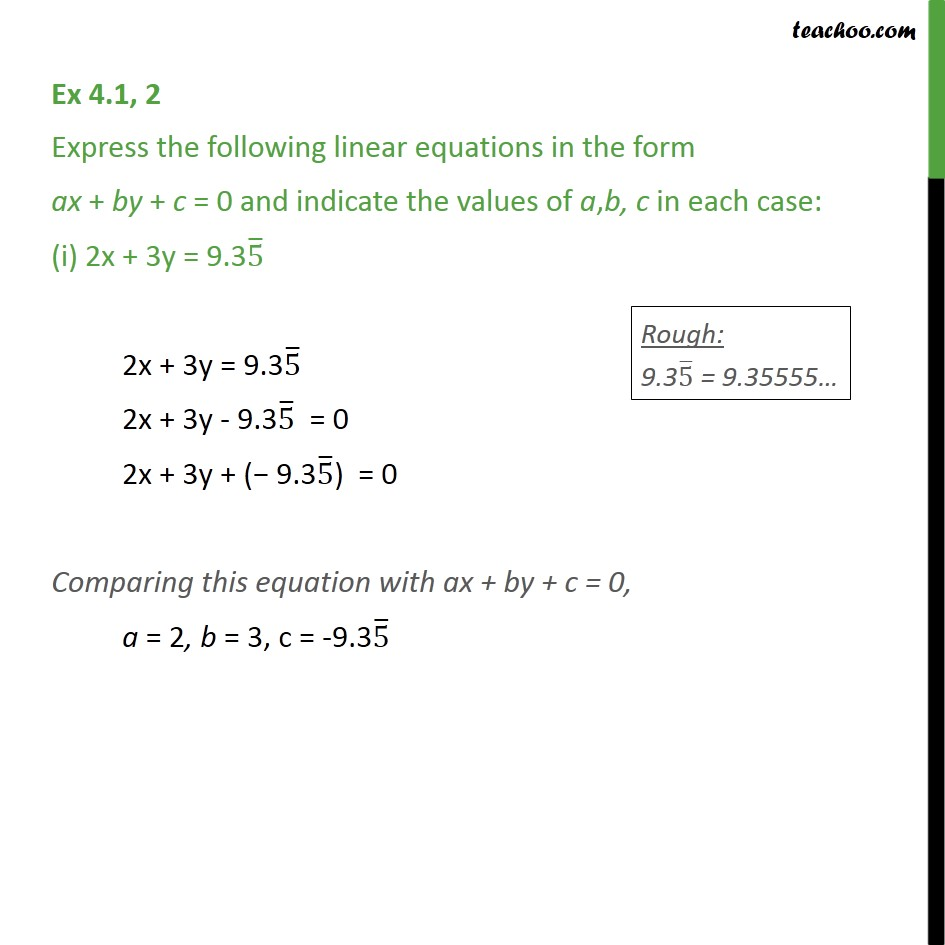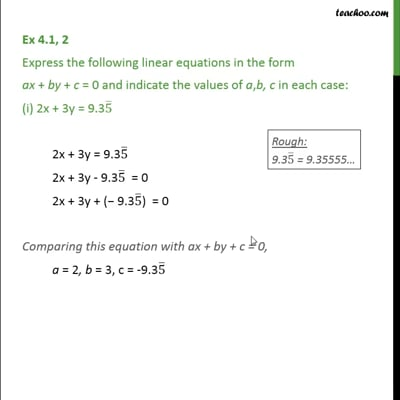Definition and General Form of Linear EquationThis video is only available for Teachoo black users

Solve all your doubts with Teachoo Black (new monthly pack available now!)

### Transcript

Ex 4.1, 2 Express the following linear equations in the form ax + by + c = 0 and indicate the values of a,b, c in each case: (i) 2x + 3y = 9.35 ̅ 2x + 3y = 9.35 ̅ 2x + 3y - 9.35 ̅ = 0 2x + 3y + (− 9.35 ̅) = 0 Comparing this equation with ax + by + c = 0, a = 2, b = 3, c = -9.35 ̅